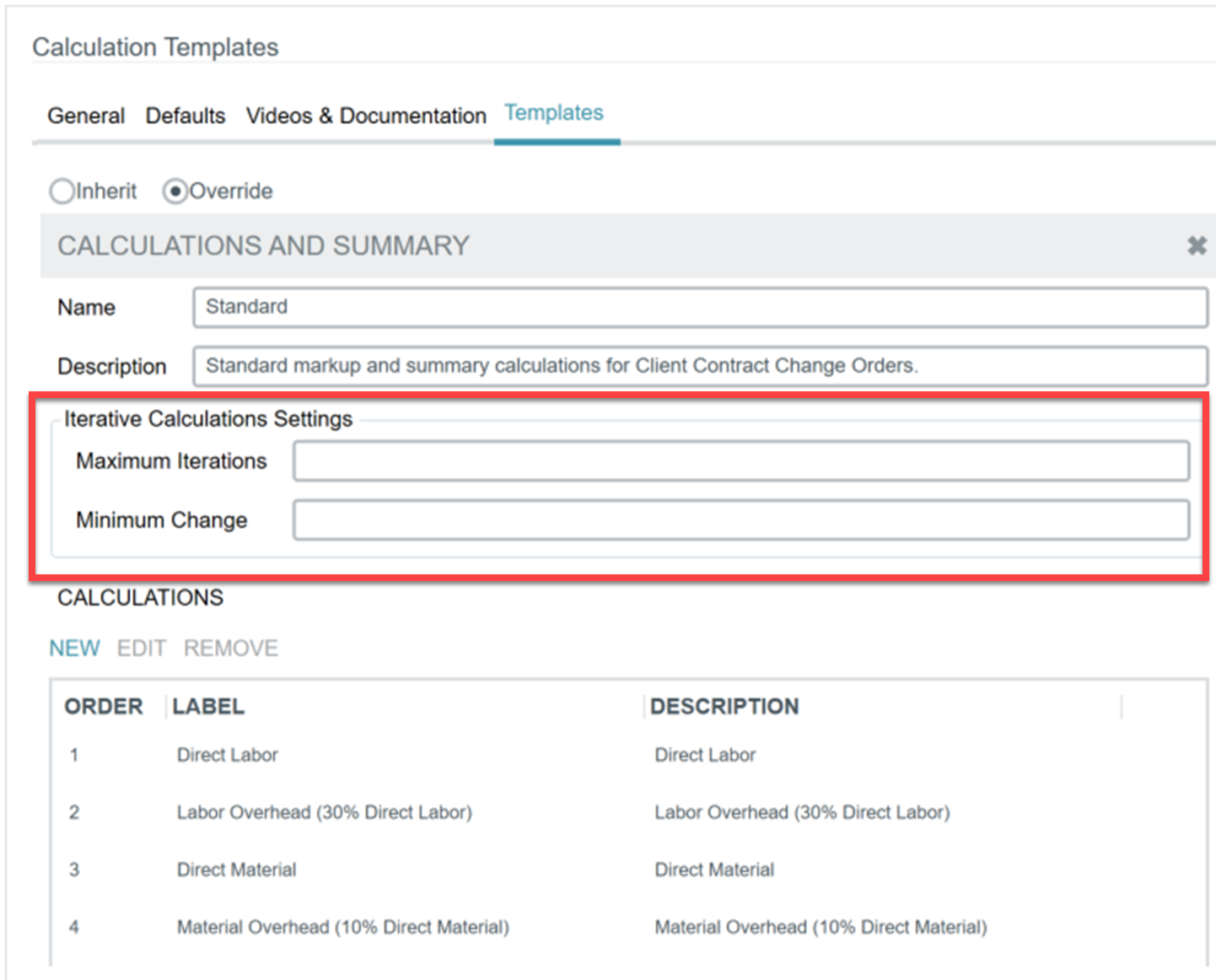An advanced feature of Kahua cost markup calculation templates is the ability to define “iterative” markup calculations. An iterative markup calculation is a calculation that has a circular relationship with other calculations defined for the calculation template. This in effect allows an organization to maximize the markup by defining the rules for iterating over a set of iterative calculations whereby for each iteration, the markup value will increase, with the difference from the prior calculation to the next calculation being added into the total. This iterative process will repeat for either a maximum number of iterations or until a minimum threshold for the change in amount between subsequent iterations is encountered, at which point the iterative processing will stop and the final amount will be set in the given calculation.

The following fields in a calculation template are used in iterative calculations:Field Name Description Maximum Iterations This field will define the maximum number of iterations performed for an iterative calculation.  The default is 50 iterations.  This field is required to be a value greater than zero if the calculation template contains iterative calculations. Minimum Change This field defines the minimum change in value between subsequent iteration calculations for a given calculation item.  The default is 0.001.  If the change in value between an iterative calculation falls below this amount, the iterative processing will terminate.  This field is required to be a value greater than zero if the calculation template contains iterative calculations.

The following fields on a stored calculation in a calculation template are used in iterative calculations:Field Name Description Calculate From Document Total If checked, this indicates that the input amount to the calculation is the current running document total.  The current running document total will be the sum of all current markup item amounts, plus the sum of a designated calculation that has the Is Direct Costs Calculation checkbox checked, which is essentially the sum of all cost items on a cost document that are considered eligible for inclusion. Minimum Change If checked, this indicates that the input amount to the calculation is the current running markup total.  The current running markup total will be the sum of all current markup item amounts.

Any calculation defined in a calculation template that has either the Calculate From Document Total or Calculate from Markup Total checked is considered an iterative calculation. In addition, any calculation which is dependent upon another calculation that has the Calculate From Document Total or Calculate From Markup Total checked is also considered an iterative calculation since the result of a dependent calculation will be recomputed each time the iterative calculation upon which it depends is recomputed.